# Figures for survey objectives

Sorry. I'm still writing the captions, so some of them are missing belowFigure 1: The QSO-galaxy angular cross-correlation function w_{qg}() for the 169 z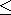0·3 EMSS QSOs and APM B_J20·5 galaxies. The dashed curve is the angular B < 20·5 galaxy auto-correlation function, w_{gg}(), derived from the APM galaxy survey (Maddox et al. 1991). The dotted lines indicate the ±2\sigma limits for the fit of w_{gg}() to w_{qg}(). The agreement between the amplitudes of the two functions implies that the clustering properties (and thus environments) of these QSOs are identical to those of galaxies.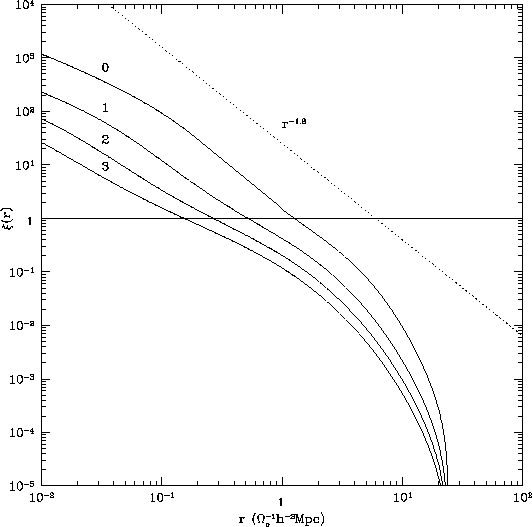Figure 2:in an unbiased,= 1, CDM model for redshifts, z = 0, 1, 2, 3, taken from Hamilton et al. (1991). A r^{-1·8} power law (dotted line) is shown for comparison.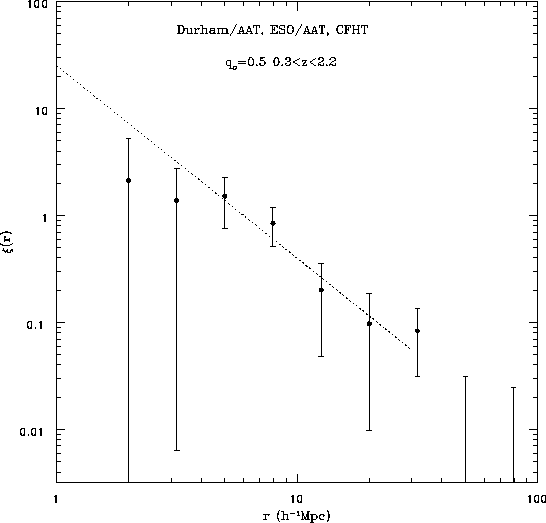Figure 3: The Durham/AAT, ESO/AAT and CFHT correlation function on small scales assuming q_0=0·5. The dotted line corresponds to(r)=(r/r_0)^{-1·8} with r_0 = 6h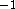Mpc (Shanks & Boyle 1994).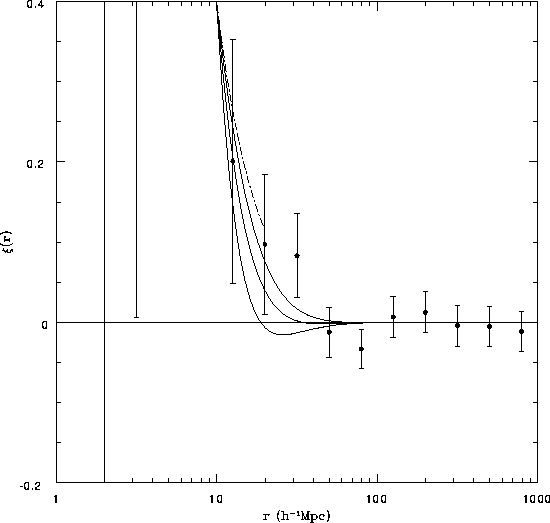Figure 4:(r) for the Durham/AAT, ESO/AAT and CFHT on large scales. The dot-dashed and representative line is a -1·8 power law with r_0 = 6hMpc, similar to the APM correlation function (Maddox et  al. (1991)). The solid lines are= 1, CDM correlation functions (from Holtzmann 1989) normalized to the detected QSO correlation function within 10hMpc, with h = 0·5 and n = 0·5, 1 and 1·5 (top to bottom), the middle solid line corresponding to standard CDM.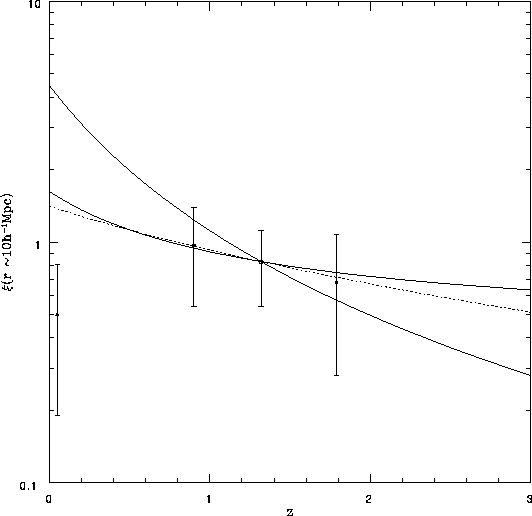Figure 5: The amplitude ofat r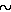10hMpc. The evolution of the amplitude ofis predicted under three simple linear evolution models which include the effect of biasing after Bardeen et al (1986). The solid lines represent= 1 with b = 1 (steeper curve) and b = 2 (shallower curve). The dotted line represents= 0·1, b = 1. The points plotted are estimates ofat approximately the 10hMpc scale taken from current surveys (see Shanks & Boyle 1994). With a ×50 increase in the sample size we shall be able to distinguish between an= 1 with 1b1·5 and the unbiased open model.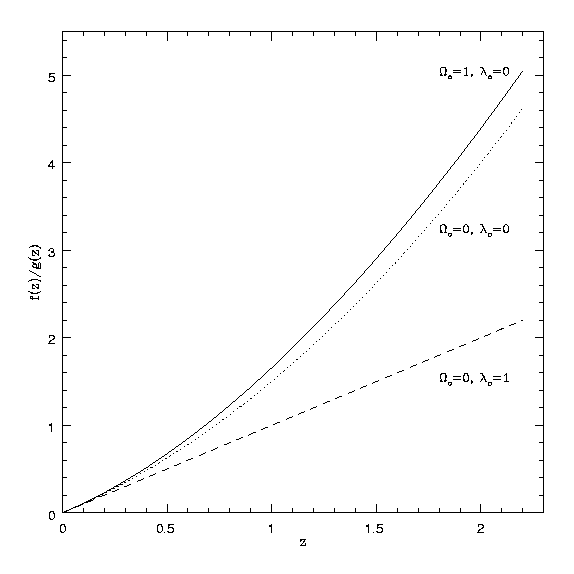Figure 6: An illustration of how the ratio of angular to radial distances varies as a function of z for different cosmological models. We plot f(z)/g(z), where the co-moving distance (r) between two QSOs separated by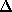z in the redshift direction andin the angular direction is given by r² = g²z² + f²². It can be seen that at high z there is a large difference between the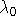=1 (=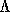c² / 3H_0²) and the=0 models (Phillipps 1994).

Robert J. Smith, rjs@astro.livjm.ac.uk